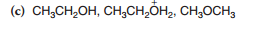# Problem: Arrange the following compounds in order of decreasing acidity:

###### Problem Details

Arrange the following compounds in order of decreasing acidity:What scientific concept do you need to know in order to solve this problem?

Our tutors have indicated that to solve this problem you will need to apply the pKa concept. You can view video lessons to learn pKa. Or if you need more pKa practice, you can also practice pKa practice problems.

What is the difficulty of this problem?

Our tutors rated the difficulty ofArrange the following compounds in order of decreasing acidi...as medium difficulty.

How long does this problem take to solve?

Our expert Organic tutor, Jonathan took 1 minute undefined to solve this problem. You can follow their steps in the video explanation above.

What textbook is this problem found in?

Our data indicates that this problem or a close variation was asked in Organic Chemistry - Solomons 10th Edition. You can also practice Organic Chemistry - Solomons 10th Edition practice problems.